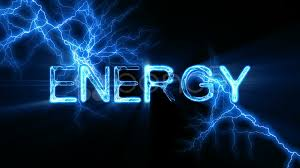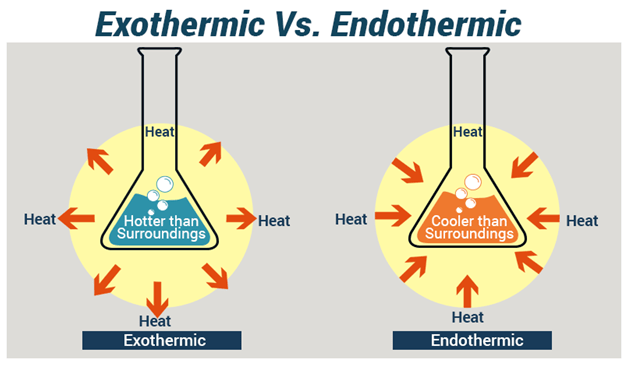# Exothermic and Endothermic Reactions

Welcome to Class !!

In today’s Chemistry class, We will be learning about Exothermic and Endothermic Reactions. We hope you enjoy the class!

CONTENT

• Heat Content (Enthalpy) of a substance.
• Types of Heat of Reactions.
• Thermodynamics: First and Second Laws.
• Entropy and Free energyENERGY

Energy is defined as the ability to do work. It exists in different forms like heat, light, sound, electrical, potentials (stored), kinetic etc.

LAWS OF CONSERVATION OF ENERGY

Energy can be changed from one form to another. The total amount of energy before and after the change remains the same. This observation is stated in the law of conservation of energy which states that energy can neither be created nor destroyed but can be changed from one form to another.

There are types of energy such as chemical energy, heat energy, and light energy.

HEAT CONTENT (ENTHALPY) OF A SUBSTANCE

Heat content or Enthalpy of a substance is the characteristic internal energy possessed by the substance, which is due to the structure and physical state of a substance. The potential energy is due to the structure while the kinetic energy is due to the physical state. Enthalpy of one substance is different from another. Total enthalpy cannot be measured but only enthalpy change. Generally, an enthalpy change (∆H) is the heat that would be exchanged with the surrounding, that is, it is the amount of energy involved in a reaction.

Thus,

Enthalpy change = Heat of products – Heat of reactants

That is, ∆H = Hproducts – Hreactants

The enthalpy change of a given reaction is always written side by side with the given equation and it may be either a positive or negative value.

Example: HCl(aq) + NaOH(aq) → NaCl(aq) + H2O(l)      ∆H = -57.3kJ

Unit of enthalpy change(∆H) is Joules(J) or kilojoules(kJ)

#### EXOTHERMIC AND ENDOTHERMIC REACTIONS

EXOTHERMIC REACTION

A chemical reaction in which heat is given off to the surrounding is known as an exothermic reaction. When an exothermic reaction occurs, heat is liberated and transferred from the chemicals to the surroundings and the temperature of the reaction mixture rises. The reaction vessel will feel hot.

Examples of exothermic reactions include:

1. The reaction between calcium oxide and water
2. The reaction between an acid and a base.
3. Combustion of fuel
4. Corrosion of metals
5. Respiration

In an exothermic reaction, the enthalpy change is negative since the heat content of the products is less than the heat content of the reactants.ENDOTHERMIC REACTION

An Endothermic reaction is a type of reaction in which heat is absorbed from the surroundings. When an endothermic reaction occurs, heat energy is absorbed and transferred from the surrounding to the reactants and the temperature of the reaction mixture falls. The reaction vessel will feel cold.

Examples of endothermic reactions are:

1. Thermal decomposition of calcium trioxocarbonate (IV)
2. Thermal dissociation of ammonium chloride
3. The action of light on silver bromide in photographic film
4. Photosynthesis in plants

In an endothermic reaction, the heat content of the product is more than the heat content of the reactant; hence the enthalpy change is positive.

ENERGY LEVEL DIAGRAMS

Energy changes can be presented by diagrams, which shows at once whether reactions are exothermic or endothermic.EVALUATION

1. What is enthalpy?
2. Giving TWO examples each, define endothermic and exothermic reactions.

HEAT OF REACTION AND CHEMICAL BONDS

During chemical reactions, chemical bonds are broken, atoms are regrouped and new bonds are formed. Bond breaking requires energy and bond-forming evolves energy. The minimum amount of energy required for bond breaking is called activation energy. While bond breaking is endothermic, bond-forming is exothermic. Thus, the heat of reaction comes from breaking and forming of chemicals bond. Heat reaction is negative [exothermic] when bond-breaking energy is less than bond-forming energy. The heat of reaction is positive [endothermic] when bond-breaking energy is more than bond-forming energy.

TYPES OF HEAT CHANGES IN CHEMICAL REACTIONS

HEAT OF FORMATION

The amount of heat evolved or absorbed when one mole of a substance is formed from its elements is known as the heat of formation [or enthalpy of formation].

The standard heat of formation of a substance (∆Hfθ) is the heat evolved or absorbed when one mole of that substance is formed from its elements under standard conditions.

For the formation of 1 mole of liquid water, the equation is

H2(g)  + 1/2O2(g)® H2O(1)          ∆Hfθ  = – 285kJmol-1

Thus, ∆Hfθ of water = – 285kJmol-1

HEAT OF NEUTRALIZATION

Neutralization is an exothermic reaction. The amount of heat evolved during a neutralization reaction in which one mole of water is formed is known as the heat of neutralization (or enthalpy of neutralization). The standard heat of neutralization ∆Hnθ  is the amount of heat evolved when 1 mole of hydrogen ions, H+, from an acid reacts with 1 mole of hydroxide ions, OH, from an alkali to form 1 mole of water under standard conditions. The heat of neutralization is also known as the heat of formation of one mole of water from its ionic components.

H+(aq)  +  OH(aq)   →  H2O(l)            ∆Hnθ  = – 57.4kJmol-1

HEAT OF COMBUSTION

A combustion reaction is always exothermic. The amount of heat evolved when one mole of a substance is burned completely in oxygen is known as the heat of combustion or enthalpy of combustion. The standard heat of combustion of a substance, ∆HCθ; is the heat evolved when one mole of the substance is burned completely in oxygen under standard conditions.

A bomb calorimeter is usually used for accurate determination of heat of combustion.

The heat of combustion can be determined from the relation below:

When the heat evolved by the burning substance is used to raise the temperature of a known mass of water, then the expression for the heat of combustion can be given as:

Where m = mass of water

C = Specific heat capacity of water

∆θ = change in temperature, that is, θ2 – θ1

HEAT OF SOLUTION

The heat of solution can be exothermic or endothermic. The heat of solution is the heat evolved or absorbed when one mole of a substance is dissolved in so much water that further dilution results in no detectable heat change.

Standard heat of solution, ∆Hsθ, is the amount of heat evolved or absorbed when 1 mole of a substance is dissolved in so much water that further dilution results in no detectable heat change at standard conditions.

EVALUATION

Define each of the following:

1. Heat of combustion
2. Heat of neutralization

### THERMODYNAMICS

Thermodynamics is the study of the relationship between heat and other forms of energy.

A system in thermodynamics is any part of the universe chosen for thermodynamics consideration, i.e. the physical and chemical phenomenon or process occurring in a given environment.  A system can be isolated, closed or open.

Surrounding is the environment in which a phenomenon or a process occurs.

The first law of thermodynamics states that energy can neither be created nor destroyed but may be converted from one form to another.

In thermodynamics, heat is represented by q and other forms of energy are referred to as work denoted by w.  The conditions or state of a chemical system is changed when:

1. Heat is evolved or absorbed
2. Work is done on or by the system

In any case, the internal energy, U, of the system is affected and it is changed.

From first law, heat is changed into internal energy of the system it may be represented by

change in internal energy = Heat absorbed by the system + Work done by the system

i.e.       U          =          q         +          w

Work done by the system is negative since this lead to a decrease in internal energy, therefore:

U          =          q         –           w

For a gaseous system,    w  =  P  V

U          =          q        –           P    V

U          =          H        –           P    V

H         =          U         –           P    V

EVALUATION

1. State the first law of thermodynamic
2. Calculate:

(a) the heat absorbed by a system when it does 72J of work and its internal energy decreases by 90J

(b) U for a gas that releases 35J of heat and has 128J of work done on it.

#### SECOND LAW OF THERMODYNAMICS

The second law of thermodynamic states that a spontaneous process occurs only if there is an increase in the entropy of a system and its surroundings

Factors which determines the spontaneously of a process are:

1. enthalpy, H: The heat content of the substances involved
2. entropy, S: The measure of the degree of disorderliness or randomness of a substance
3. free energy G: The energy which is available for doing work.

ENTROPY (S)

Entropy is the measure of the degree of disorderliness or randomness of a system. The standard entropy change (∆Sθ) is a state function because it depends on the initial and final state of the system. That is:

∆Sθ = Sθproducts – Sθreactants

The S.Iunit of is JK-1mol-1

Entropy increases from solid to liquid to gaseous state because as you go from solid to liquid to a gaseous state, randomness increases, that is; ∆Sθ tends to positive.

For a reversible process at constant temperature,

S      =          H/T

When ∆S is positive, there is an increase in entropy.  When ∆S is negative there is a decrease in the entropy of a system.

GIBB’S FREE ENERGY

The free energy of a system is the energy which is available for doing work in the system; that is, the driving force that brings about a chemical change.

The standard free energy change (∆Gθ) is a state function because it depends on the initial and final state of the system. That is:

∆Gθ = Gθproducts – Gθreactants

Free energy takes into account the effect of the enthalpy and entropy factors as represented in the equation below:

G = H-TS

For a change at constant temperature,

G =     H – T    S

NOTE:

1. When G is negative, the reaction is spontaneous or feasible.
2. When G is positive, the reaction is not spontaneous, unless the resultant effect of both      H and    S leads to a net decrease in     G
3. When    G is zero, the system is in equilibrium

Example: The reaction:  C(s) +  O2(g)                 CO2(g)

is carried out at a temperature of 57oC.  If the enthalpy change is -500J and the entropy change is +15J.Calculate the free energy change

Solution:

G =         H  – T   S

=       -5000  – (57 + 273)  x  (+15)

=       -5000  – 330 x 15

=       -5000  – (+4950)

=       -5000  – 4950

=       -9950J or -9.950kJ

New School Chemistry for SSS by O.Y. Ababio (6th edition) pages 230- 242

WEEKEND ASSIGNMENT

1. State THREE factors that determine the spontaneity of a chemical reaction.
2. 92g of ethanol raised the temperature of 100g of water from 298K to 312.3K when burned completely. What is the heat of combustion of ethanol?

We have come to the end of this class. We do hope you enjoyed the class?

Should you have any further question, feel free to ask in the comment section below and trust us to respond as soon as possible.

In our next class, we will be learning about Chemical Equilibrium. We are very much eager to meet you there.

Are you a Parent? Share your quick opinion and win free 2-month Premium Subscription

Don`t copy text!